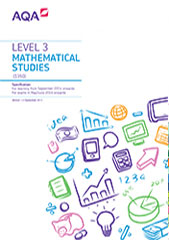# Subject content

Mathematics is, inherently, a sequential subject. There is a progression of material through all levels at which the subject is studied. It is assumed that students will already have confidence and competence in the content presented in standard type within the GCSE mathematics criteria. Students will make use of elements of this content when addressing problems within this Level 3 Certificate Mathematical Studies specification but this is not explicitly set out in subject content. This Level 3 Certificate Mathematical Studies specification aims to build on the knowledge, understanding and skills established in GCSE mathematics.

Subject content that is expected to be used throughout this specification is listed below:

• Knowledge and use of the formula . It is also expected that students will be able to find the gradient of a straight line connecting two different points.

• It is expected that spreadsheets and tables will be used throughout the teaching of this Level 3 Certificate Mathematical Studies specification. Spreadsheet formulae will include:

• “=A1+A2+A3” to sum values in cells
• “=2*B3” to multiply a value in a given cell
• “=SUM(A1:A10)”
• Knowledge and use of the formulae for the circumference and the area of circle.

• Knowledge and use of the formulae for the perimeter of 2-D shapes, their areas and for calculating fractional areas of circles and composite shapes.

• Knowledge and use of the mathematical content of analysis of data and maths for personal finance elements.

• The ability to calculate surface areas of spheres, cones, pyramids and composite solids, including the application of the concepts of similarity including lengths in similar figures and Pythagoras’ theorem applied to 2-D and 3-D figures.

• Students will make use of elements of the content from paper 1 when addressing problems within each paper 2.